Study Materials

# NCERT Solutions for Class 9th Mathematics

Page 6 of 9

## Chapter 13. Surface Areas and Volumes

### Exercise 13.6

EXERCISE 13.6

Assume π = 22/7, unless stated otherwise.

Q1. The circumference of the base of a cylindrical vessel is 132 cm and its height is 25 cm. How many litres of water can it hold? (1000 cm3 = 1 l)

Solution:

Circumference of cylinder = 132 cm

Height = 25 cm

2πr = 132 cm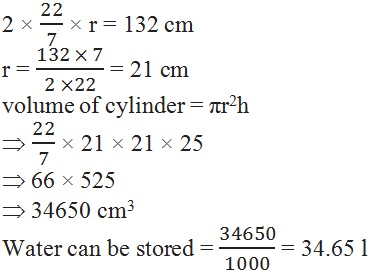Q2. The inner diameter of a cylindrical wooden pipe is 24 cm and its outer diameter is 28 cm. The length of the pipe is 35 cm. Find the mass of the pipe, if 1 cm3 of wood has a mass of 0.6 g.

Solution:

(i) volume of inner cylinder = πr2h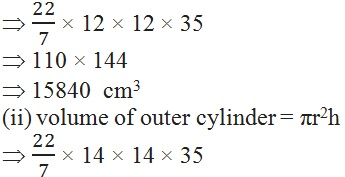110 × 196

21560 cm3

Total volume = v1 + v2

⇒ 15840 + 21560 cm3

37400 cm3

Mass = 37400 × 0.6

22440

Q3. A soft drink is available in two packs – (i) a tin can with a rectangular base of length 5 cm and width 4 cm, having a height of 15 cm and (ii) a plastic cylinder with circular base of diameter 7 cm and height 10 cm. Which container has greater capacity and by how much?

Solution:

Length = 5cm, breadth = 4cm, height = 15cm

Diameter = 7cm, height = 10cm, r = 3.5cm

Volume of cuboid = l × b × h

5 × 4 × 15 cm

20 × 15 cm

300 cm3

Volume of cylinder = πr2h

× 3.5 × 3.5 × 10

22 × 5 × 3.5

110 × 3.5

385 cm3

Capacity of both

300 cm=  385 cm3

385 – 300

85 cm3

Q4. If the lateral surface of a cylinder is 94.2 cm2 and its height is 5 cm, then find

(i) radius of its base       (ii) its volume. (Use  = 3.14)

Solution:

Height = 5 cm

Lateral surface area of the cylinder = 2πrh

2 × 3.14 × r × 5 = 94.2 cm2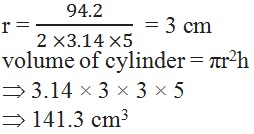Q5. It costs 2200 to paint the inner curved surface of a cylindrical vessel 10 m deep. If the cost of painting is at the rate of 20 per m2, find

(i) inner curved surface area of the vessel,

(iii) capacity of the vessel.

Solution:

Cost = Rs. 2200

Height = 10 m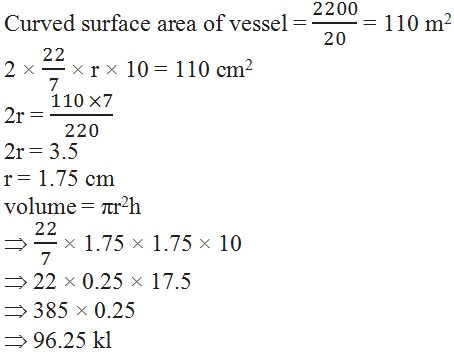Q6. The capacity of a closed cylindrical vessel of height 1 m is 15.4 litres. How many square metres of metal sheet would be needed to make it?

Solution:

Height = 1 m

Capacity = 15.4 l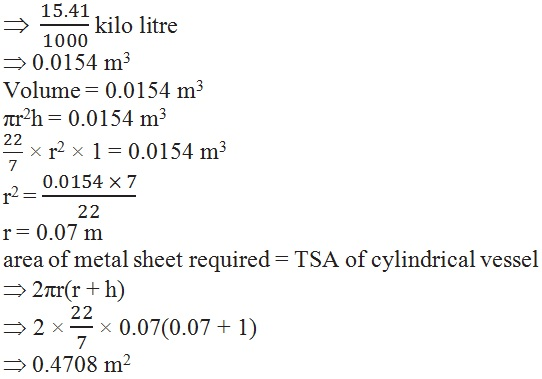Q7. A lead pencil consists of a cylinder of wood with a solid cylinder of graphite filled in the interior. The diameter of the pencil is 7 mm and the diameter of the graphite is 1 mm. If the length of the pencil is 14 cm, find the volume of the wood and that of the graphite.

Solution:Q8. A patient in a hospital is given soup daily in a cylindrical bowl of diameter 7 cm. If the bowl is filled with soup to a height of 4 cm, how much soup the hospital has to prepare daily to serve 250 patients?

Solution: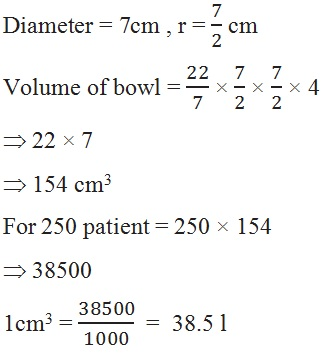Page 6 of 9

Chapter Contents: